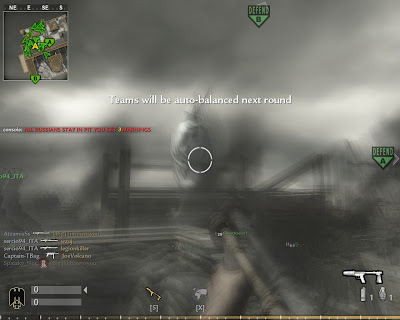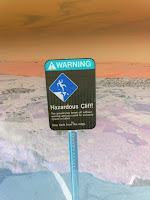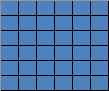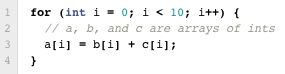## Sunday, January 15, 2012

### Parallel Image Processing

The emergence of AJAX, Facebook, Youtube, iPhone, iPad, and cloud computing has created the perfect storm for the greatest societal changes in history-- social media.  We have become crazed consumers of content on the web.  This blog post will explain the basics behind a digital image.

Videos are made up of frames.  Think of the term 'frames per second'.  A frame is just a still image.  When you flash a bunch of frames at a rate of thirty per second, the human eye perceives it as video.  Each frame can be edited individually to achieve various visual effects such as inversion or smearing.This frame uses smear + black-and-white image filters.

Let's break it down a bit further.  The first question is-- what is an image?  An image is made up of a bunch of pixels.  For example, a 100x100 resolution image has 100 rows and 100 columns for a total of 10,000 pixels.

Breaking it down even further, the second question is-- what is a pixel?  A pixel is a dot of color.  A pixel is a mixture of three different colors in the RGB model-- red, green, and blue.  Most images use 24-bit pixels with red, green, and blue taking 8 bits each.  This allows for a depth range between 0 and 255 (8 bits unsigned can go up to 255).  A depth range of 256 means that the color can have 256 different shades of intensity.  Since the final pixel color is the result of mixing red, green, and blue together, the total number of possible pixel colors is 256*256*256 = 16,777,216.  Many R,G,B charts like this can be found online.

Let's recap.  A video is made from images.  An image is made from pixels.  A pixel is made from three R,G,B values.  Now that we've broken down the process completely, it is easy to understand that image processing boils down to the manipulation of individual pixels via their R,G,B values.  For example, we can transform a black pixel into a white pixel by changing its R,G,B value from (0,0,0) to (255,255,255).

We can apply a visual effect to an entire image by using something called an image filter.  It is a fancy term for describing an algorithm that manipulates a set of pixels to achieve a certain effect.  To show what goes on behind the scenes in actual code, I wrote two Java programs that take an image file as input, applies an invert/oil filter, then outputs the new image file.  The full source code is available at my GitHub project site.OriginalInvertedOiled

The algorithm for inverting an image is

• For each pixel (x,y) in the original image with (R,G,B) values:
• Subtract 255 from the value of R
• Do the same for the G and B values
• For example, the inversion of a white pixel (255,255,255) is a black pixel (0,0,0)The complete source code is available here in ConcurrentInvert.java

The algorithm for oiling an image is
• For each pixel (x,y) in the original image with (R,G,B) values:
• Replace the R value by the most common R value among all pixels with x-coordinates between (x - radius) and (x + radius) and with y-coordinates between (y - radius) and (y + radius)
• Do the same for the G and B values
• For example, if radius = 1, one must find the most common R,G,B values among 9 pixels (in a 3x3 grid).  If radius = 2, one must find the most common R,G,B values values among 25 pixels (in a 5x5 grid)The complete source code is available here in ConcurrentOil.java

Inverting an image has a time complexity of O(n) so the program runs pretty quickly.  However, oiling an image has a time complexity of O((2n+1)*(2n+1)*n) = O(4n^3 + 4n^2 + n) so it runs slower by several orders of magnitude.

Luckily, we can achieve a big speedup by using parallelization, also known as multi-threading.  The concept is simple.  We create additional threads and split the pixels as evenly as possible across all cores.Workload on a single threadWorkload on two threads

The caveat is that parallelization only works when the order of execution does not matter, or when the result of a computation does not depend on the result of another computation.  For example, the following piece of code can be parallelizedThe order in which this for loop executes does not matter.  We could parallelize this computation across 2 threads by having the first thread compute iterations i=0 to i=4, and the second thread compute iterations i=5 to i=9.

whereas the next piece of code cannot be parallelizedThe order in which this for loop executes does matter.  We must compute iteration i=2 before i=3, i=3 before i=4, and so on.

Test results show that the algorithm for inverting and oiling an image is perfectly parallelizable and scalable to an infinite number of cores.Inverting the cliff image above is twice as fast on 2 cores vs 1 coreOiling the cliff image above is twice as fast on 2 cores vs 1 core

The concepts presented here form the basic backbone of all digital image and video processing in programs like Photoshop, Final Cut Pro, and even the camera in your smartphone.  In this age where image and video sharing is an epidemic on social media sites like Facebook and Youtube, I hope that readers have gained a better appreciation for how images and videos work.